# Module 14 Recursion Module 14 Recursion Rosen 5

• Slides: 30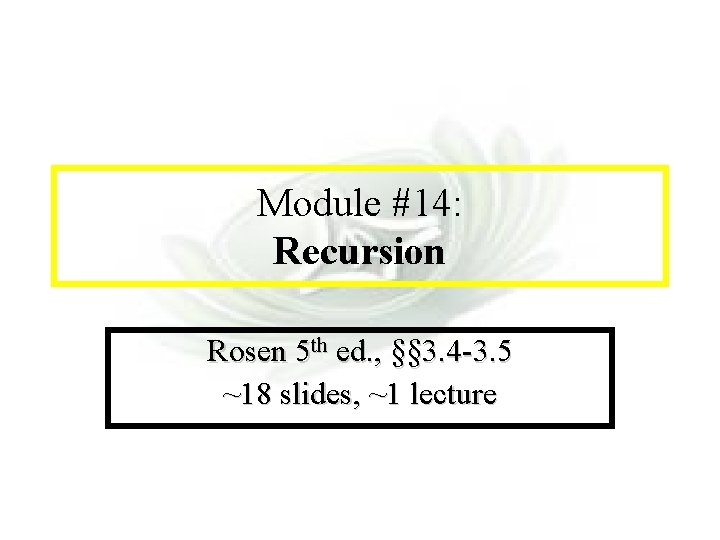Module #14 - Recursion Module #14: Recursion Rosen 5 th ed. , §§ 3. 4 -3. 5 ~18 slides, ~1 lecture 2020/9/9 (c)2001 -2003, Michael P. Frank 1Module #14 - Recursion § 3. 4: Recursive Definitions • In induction, we prove all members of an infinite set have some property P by proving the truth for larger members in terms of that of smaller members. • In recursive definitions, we similarly define a function, a predicate, or a set over an infinite number of elements by defining the function or predicate value or set-membership of larger elements in terms of that of smaller ones. 2020/9/9 (c)2001 -2003, Michael P. Frank 2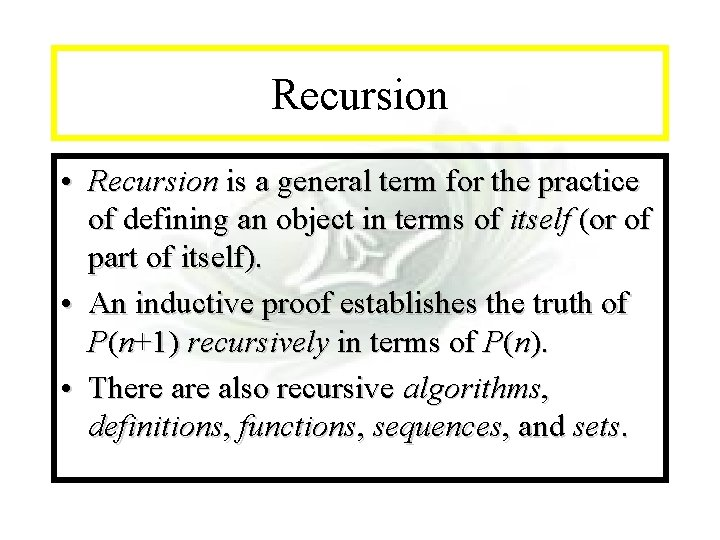Module #14 - Recursion • Recursion is a general term for the practice of defining an object in terms of itself (or of part of itself). • An inductive proof establishes the truth of P(n+1) recursively in terms of P(n). • There also recursive algorithms, definitions, functions, sequences, and sets. 2020/9/9 (c)2001 -2003, Michael P. Frank 3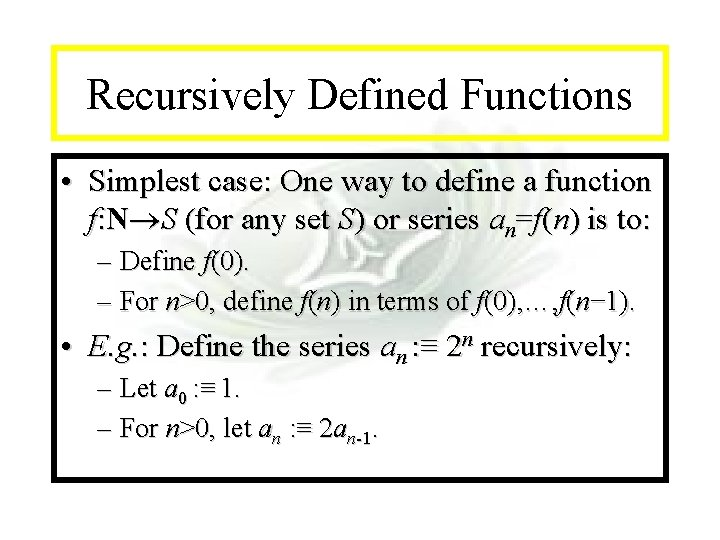Module #14 - Recursion Recursively Defined Functions • Simplest case: One way to define a function f: N S (for any set S) or series an=f(n) is to: – Define f(0). – For n>0, define f(n) in terms of f(0), …, f(n− 1). • E. g. : Define the series an : ≡ 2 n recursively: – Let a 0 : ≡ 1. – For n>0, let an : ≡ 2 an-1. 2020/9/9 (c)2001 -2003, Michael P. Frank 4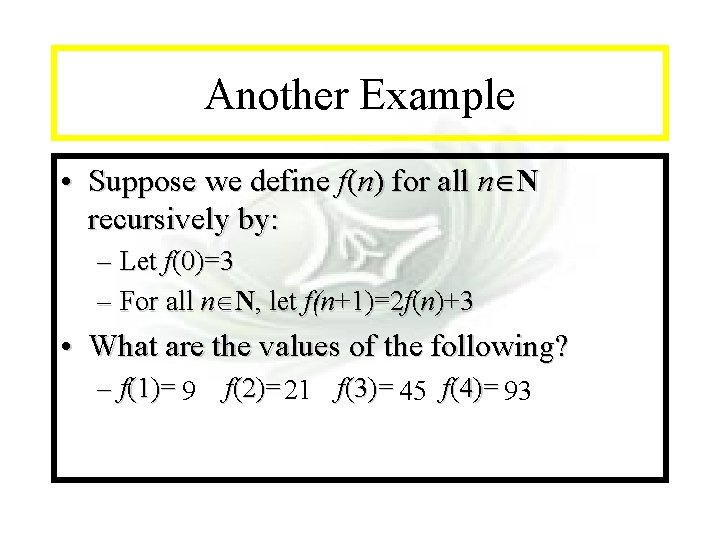Module #14 - Recursion Another Example • Suppose we define f(n) for all n N recursively by: – Let f(0)=3 – For all n N, let f(n+1)=2 f(n)+3 • What are the values of the following? – f(1)= 9 2020/9/9 f(2)= 21 f(3)= 45 f(4)= 93 (c)2001 -2003, Michael P. Frank 5Module #14 - Recursion Recursive definition of Factorial • Give an inductive definition of the factorial function F(n) : ≡ n! : ≡ 2 3 … n. – Base case: F(0) : ≡ 1 – Recursive part: F(n) : ≡ n F(n-1). • • • 2020/9/9 F(1)=1 F(2)=2 F(3)=6 (c)2001 -2003, Michael P. Frank 6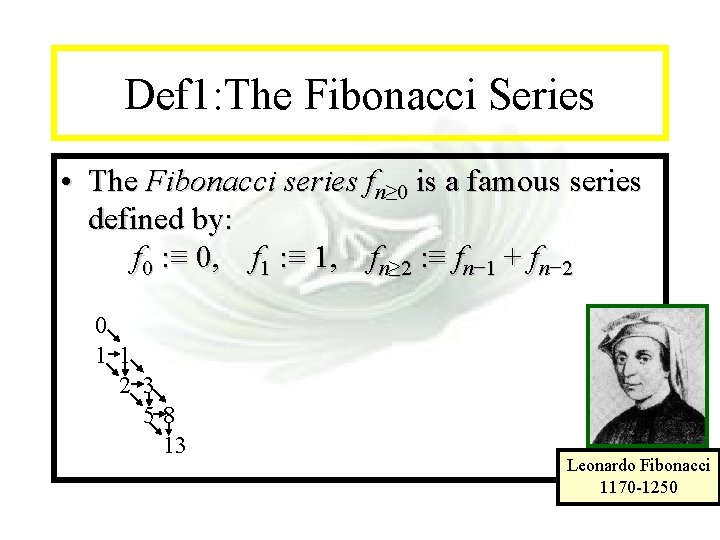Module #14 - Recursion Def 1: The Fibonacci Series • The Fibonacci series fn≥ 0 is a famous series defined by: f 0 : ≡ 0, f 1 : ≡ 1, fn≥ 2 : ≡ fn− 1 + fn− 2 0 1 1 2 3 58 13 2020/9/9 Leonardo Fibonacci 1170 -1250 (c)2001 -2003, Michael P. Frank 7Module #14 - Recursion Inductive Proof about Fib. series Implicitly for all n N • Theorem: fn < 2 n. • Proof: By induction. Note use of 0 Base cases: f 0 = 0 < 2 = 1 base cases of f 1 = 1 < 21 = 2 recursive def’n. Inductive step: Use 2 nd principle of induction (strong induction). Assume k<n, fk < 2 k. Then fn = fn− 1 + fn− 2 is < 2 n− 1 + 2 n− 2 < 2 n− 1 + 2 n− 1 = 2 n. ■ 2020/9/9 (c)2001 -2003, Michael P. Frank 8Module #14 - Recursion Inductive Proof about Fib. series • Theorem: whenever n 3, fn > an-2, where • Proof: • Basic step: a<2= f 3, a 2= = f 4 • Inductive step : Assume fj > aj-2 is true with , where. • Since a 2= a+1, ak-1= a 2．ak-3=(a+1) ak-3= ak-2+ ak-3 • fk+1= fk+ fk-1> ak-2+ ak-3= ak-1 2020/9/9 (c)2001 -2003, Michael P. Frank 9Module #14 - Recursion LAME’S THEOREM • Let a and b be positive integers with. Then the number of divisions used by Euclidean algorithm to find gcd(a, b) is less than or equal to 5 times the number of decimal digits in b. Proof: 2020/9/9 (c)2001 -2003, Michael P. Frank 10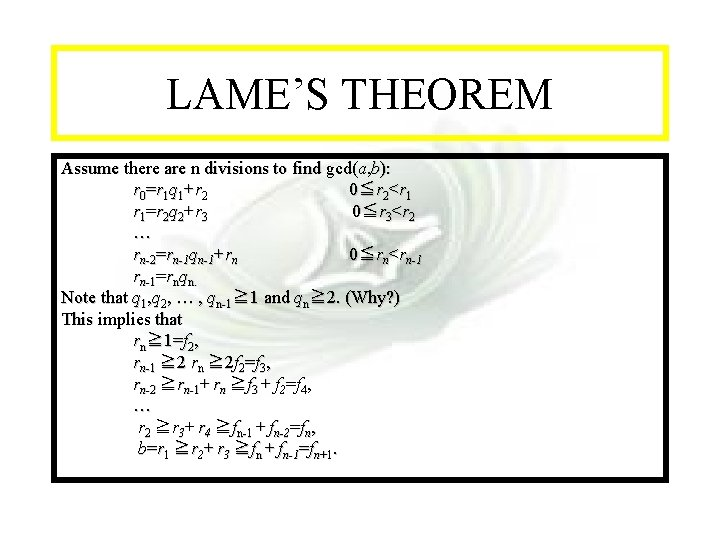Module #14 - Recursion LAME’S THEOREM Assume there are n divisions to find gcd(a, b): r 0=r 1 q 1+r 2 0≦r 2<r 1 r 1=r 2 q 2+r 3 0≦r 3<r 2 … rn-2=rn-1 qn-1+rn 0≦rn<rn-1=rnqn. Note that q 1, q 2, … , qn-1≧ 1 and qn≧ 2. (Why? ) This implies that rn≧ 1=f 2, rn-1 ≧ 2 rn ≧ 2 f 2=f 3, rn-2 ≧rn-1+ rn ≧f 3 + f 2=f 4, … r 2 ≧r 3+ r 4 ≧fn-1 + fn-2=fn, b=r 1 ≧r 2+ r 3 ≧fn + fn-1=fn+1. 2020/9/9 (c)2001 -2003, Michael P. Frank 11Module #14 - Recursion LAME’S THEOREM From Fibonacci series. We have fn+1 > an-1 for n>2. ( It follows that b > an-1. Since Hence Suppose b has k decimal digits. Then This finishes the proof. 2020/9/9 (c)2001 -2003, Michael P. Frank ) , . 12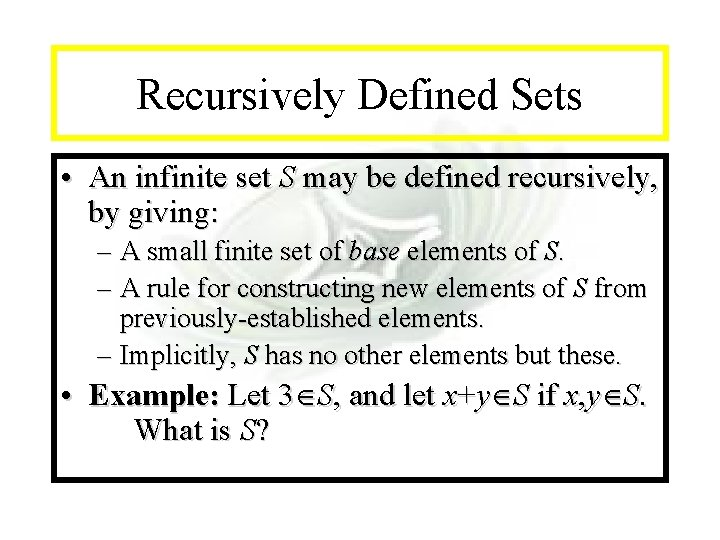Module #14 - Recursion Recursively Defined Sets • An infinite set S may be defined recursively, by giving: – A small finite set of base elements of S. – A rule for constructing new elements of S from previously-established elements. – Implicitly, S has no other elements but these. • Example: Let 3 S, and let x+y S if x, y S. What is S? 2020/9/9 (c)2001 -2003, Michael P. Frank 13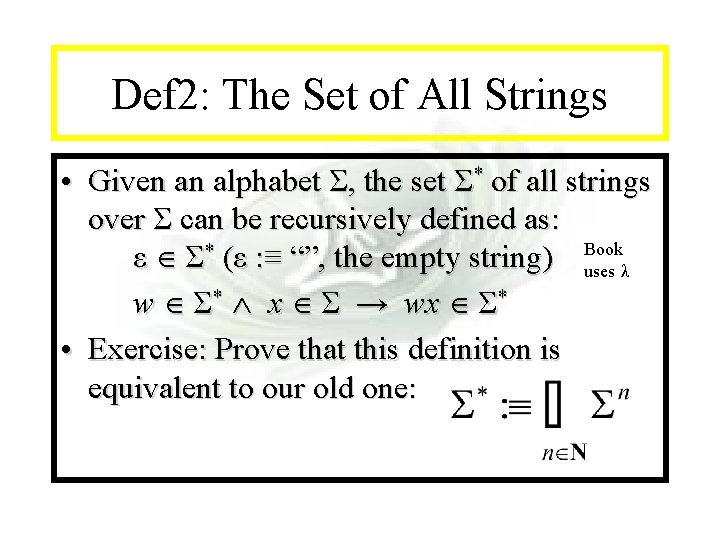Module #14 - Recursion Def 2: The Set of All Strings • Given an alphabet Σ, the set Σ* of all strings over Σ can be recursively defined as: ε Σ* (ε : ≡ “”, the empty string) Book uses λ w Σ* x Σ → wx Σ* • Exercise: Prove that this definition is equivalent to our old one: 2020/9/9 (c)2001 -2003, Michael P. Frank 14Module #14 - Recursion Def 3: String Concatenation • Let Σ be a set of symbols and Σ* the set of strings over Σ, the concatenation of two strings can be recursively defined as: Basic step: if w Σ*, then w. ε = w, ε is the empty string Recursive step: if w 1 Σ* w 2 Σ* x Σ, then w 1. (w 2 x) = (w 1. w 2 )x 2020/9/9 (c)2001 -2003, Michael P. Frank 15Module #14 - Recursion Def 4: Rooted Trees • Basis Step: A single vertex is a rooted tree. • Recursive Step: Then the graph formed by starting with a root and adding an edge from r to each of r 1, r 2, …, rn, is also a rooted tree. Where T 1, T 2, …, Tn are rooted trees with roots r 1, r 2, …, rn respectively. 2020/9/9 (c)2001 -2003, Michael P. Frank 16Module #14 - Recursion Def 5: Extended Binary Trees • Basis Step: f is an extended binary tree. • Recursive Step: If T 1 and T 2 are extended binary trees, there is an extended binary tree denoted by , consisting of a root r together with edges connecting the root to each of the roots of the left subtree T 1 and the right subtree T 2 when these trees are nonempty. 2020/9/9 (c)2001 -2003, Michael P. Frank 17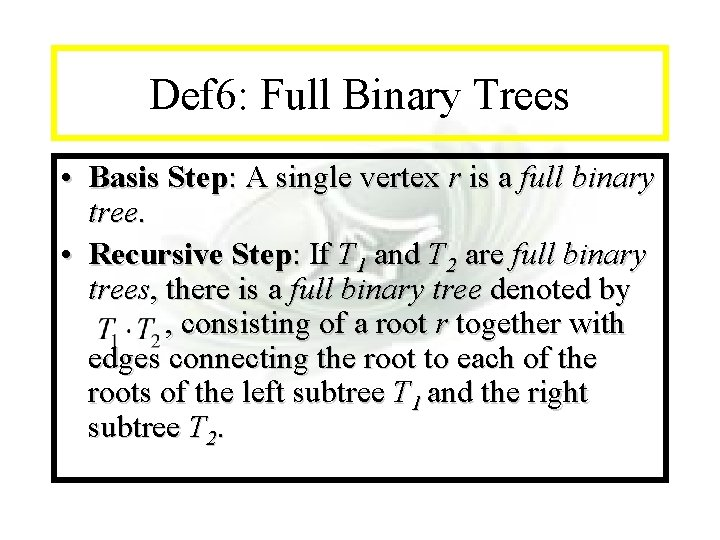Module #14 - Recursion Def 6: Full Binary Trees • Basis Step: A single vertex r is a full binary tree. • Recursive Step: If T 1 and T 2 are full binary trees, there is a full binary tree denoted by , consisting of a root r together with edges connecting the root to each of the roots of the left subtree T 1 and the right subtree T 2. 2020/9/9 (c)2001 -2003, Michael P. Frank 18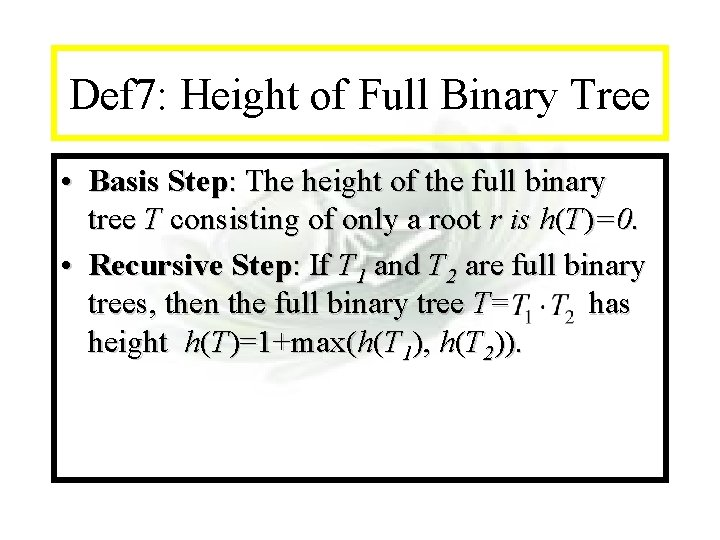Module #14 - Recursion Def 7: Height of Full Binary Tree • Basis Step: The height of the full binary tree T consisting of only a root r is h(T)=0. • Recursive Step: If T 1 and T 2 are full binary trees, then the full binary tree T= has height h(T)=1+max(h(T 1), h(T 2)). 2020/9/9 (c)2001 -2003, Michael P. Frank 19Module #14 - Recursion Recursive Algorithms (§ 3. 5) • Recursive definitions can be used to describe algorithms as well as functions and sets. • Example: A procedure to compute an. procedure power(a≠ 0: real, n N) if n = 0 then return 1 else return a · power(a, n− 1) 2020/9/9 (c)2001 -2003, Michael P. Frank 20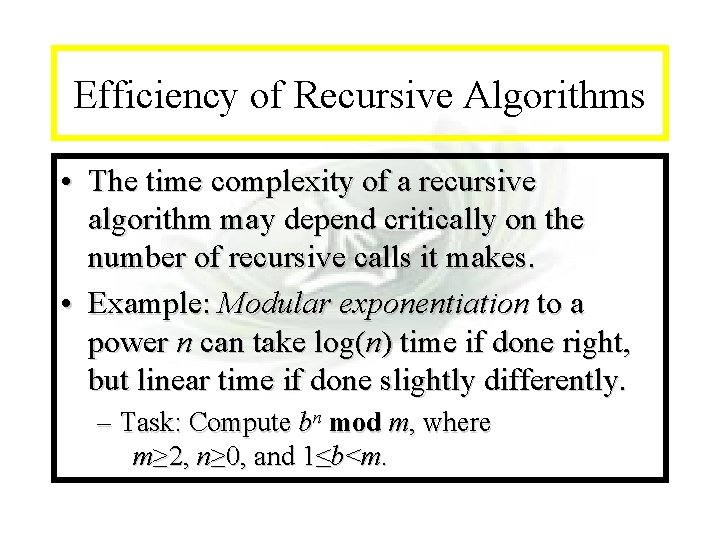Module #14 - Recursion Efficiency of Recursive Algorithms • The time complexity of a recursive algorithm may depend critically on the number of recursive calls it makes. • Example: Modular exponentiation to a power n can take log(n) time if done right, but linear time if done slightly differently. – Task: Compute bn mod m, where m≥ 2, n≥ 0, and 1≤b<m. 2020/9/9 (c)2001 -2003, Michael P. Frank 21Module #14 - Recursion Modular Exponentiation Alg. #1 Uses the fact that bn = b·bn− 1 and that x·y mod m = x·(y mod m) mod m. (Prove the latter theorem at home. ) procedure mpower(b≥ 1, n≥ 0, m>b N) {Returns bn mod m. } if n=0 then return 1 else return (b·mpower(b, n− 1, m)) mod m Note this algorithm takes Θ(n) steps! 2020/9/9 (c)2001 -2003, Michael P. Frank 22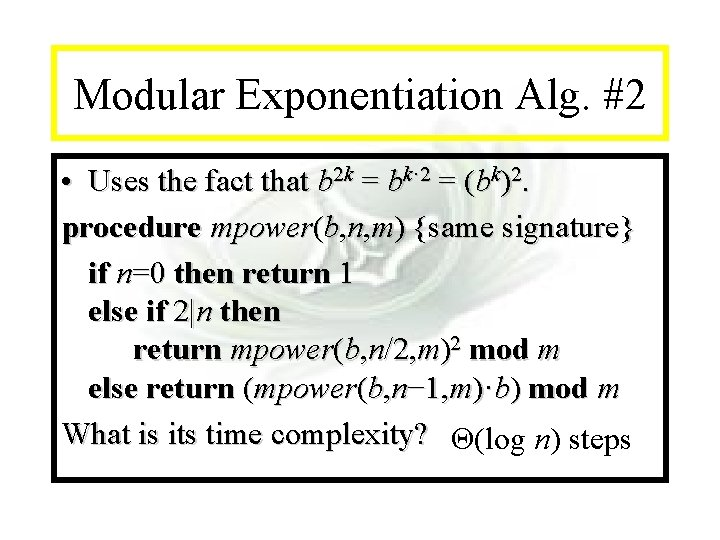Module #14 - Recursion Modular Exponentiation Alg. #2 • Uses the fact that b 2 k = bk· 2 = (bk)2. procedure mpower(b, n, m) {same signature} if n=0 then return 1 else if 2|n then return mpower(b, n/2, m)2 mod m else return (mpower(b, n− 1, m)·b) mod m What is its time complexity? Θ(log n) steps 2020/9/9 (c)2001 -2003, Michael P. Frank 23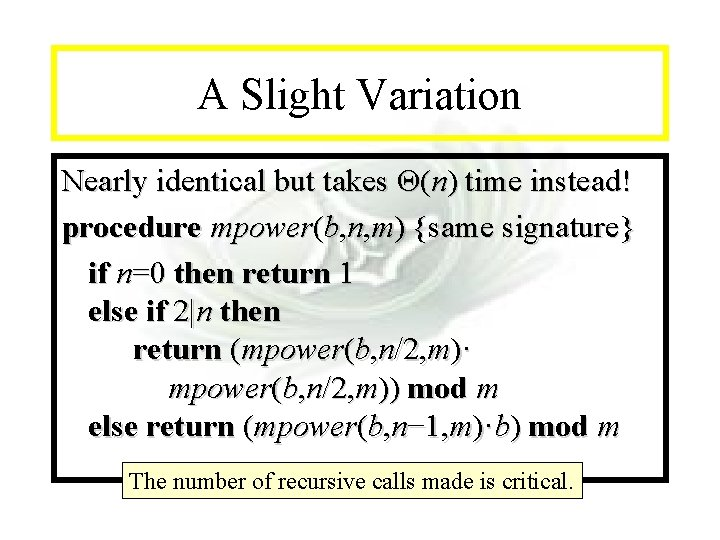Module #14 - Recursion A Slight Variation Nearly identical but takes Θ(n) time instead! procedure mpower(b, n, m) {same signature} if n=0 then return 1 else if 2|n then return (mpower(b, n/2, m)· mpower(b, n/2, m)) mod m else return (mpower(b, n− 1, m)·b) mod m The number of recursive calls made is critical. 2020/9/9 (c)2001 -2003, Michael P. Frank 24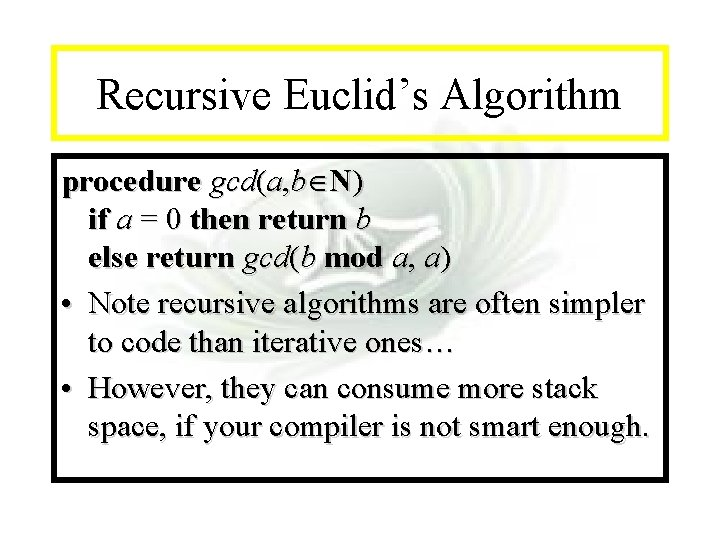Module #14 - Recursion Recursive Euclid’s Algorithm procedure gcd(a, b N) if a = 0 then return b else return gcd(b mod a, a) • Note recursive algorithms are often simpler to code than iterative ones… • However, they can consume more stack space, if your compiler is not smart enough. 2020/9/9 (c)2001 -2003, Michael P. Frank 25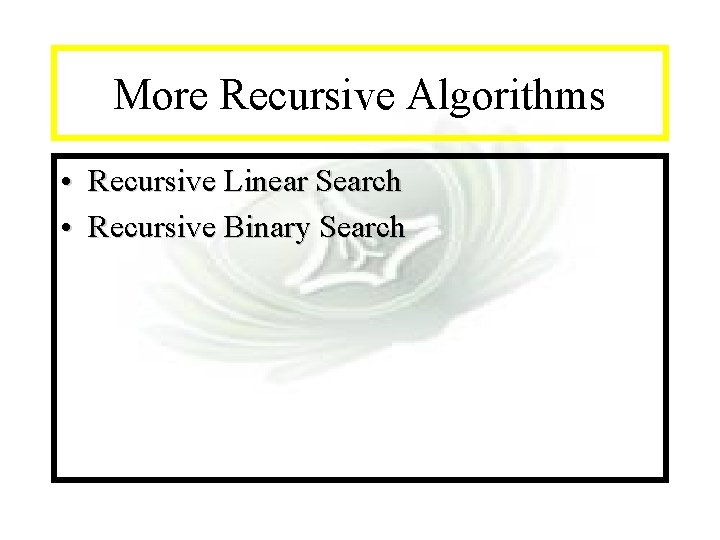Module #14 - Recursion More Recursive Algorithms • Recursive Linear Search • Recursive Binary Search 2020/9/9 (c)2001 -2003, Michael P. Frank 26Module #14 - Recursion and Iteration • Recursive Factorial vs. Iterative Factorial • Recursive Fibonacci vs. Iterative Fibonacci 2020/9/9 (c)2001 -2003, Michael P. Frank 27Module #14 - Recursion Merge Sort procedure sort(L = 1, …, n) if n>1 then m : = n/2 {this is rough ½-way point} L : = merge(sort( 1, …, m), sort( m+1, …, n)) return L • The merge takes Θ(n) steps, and merge-sort takes Θ(n log n). 2020/9/9 (c)2001 -2003, Michael P. Frank 28Module #14 - Recursion Merge Routine procedure merge(A, B: sorted lists) L = empty list i: =0, j: =0, k: =0 while ((i<|A|) (j<|B|)) {|A| is length of A} if i=|A| then Lk : = Bj; j : = j + 1 else if j=|B| then Lk : = Ai; i : = i + 1 else if Ai < Bj then Lk : = Ai; i : = i + 1 else Lk : = Bj; j : = j + 1 k : = k+1 return L Takes Θ(|A|+|B|) time 2020/9/9 (c)2001 -2003, Michael P. Frank 29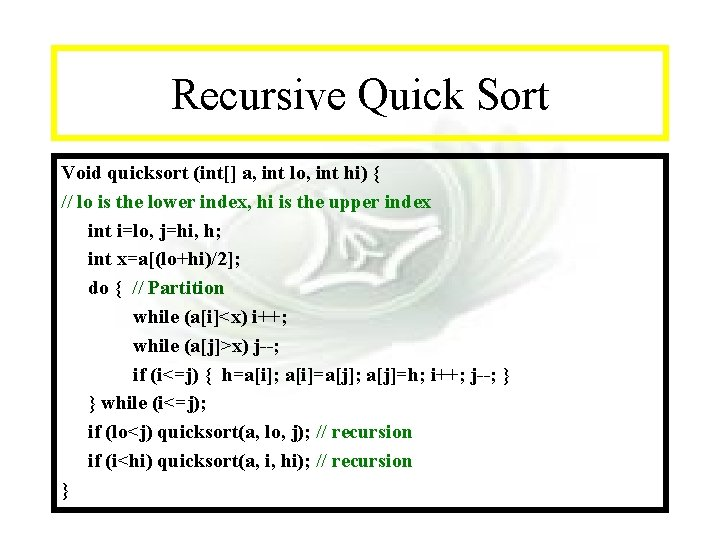Module #14 - Recursion Recursive Quick Sort Void quicksort (int[] a, int lo, int hi) { // lo is the lower index, hi is the upper index int i=lo, j=hi, h; int x=a[(lo+hi)/2]; do { // Partition while (a[i]<x) i++; while (a[j]>x) j--; if (i<=j) { h=a[i]; a[i]=a[j]; a[j]=h; i++; j--; } } while (i<=j); if (lo<j) quicksort(a, lo, j); // recursion if (i<hi) quicksort(a, i, hi); // recursion } 2020/9/9 (c)2001 -2003, Michael P. Frank 30